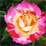# Points contained in envelopes that cross date-line - java

3268
1
Jump to solution
04-20-2015 04:57 PMNew Contributor III

I'm using the ESRI Geometry Java API https://github.com/Esri/geometry-api-java

I want to construct an envelope and see if it contains certain points. It's possible the envelope crosses the International date line.

Here is some sample code I've tried:

``    double lon = Double.parseDouble("179.99");     double lat = Double.parseDouble("0");    Point pt = new Point(lon, lat);    double minlon = Double.parseDouble("170");    double minlat = Double.parseDouble("-20");    double maxlon = Double.parseDouble("-170");    double maxlat = Double.parseDouble("20");     Envelope ev = new Envelope(minlon, minlat, maxlon, maxlat);     SpatialReference sr = SpatialReference.create(4326); // decimal degrees         boolean test = GeometryEngine.contains(ev, pt, sr);     System.out.println(GeometryEngine.geometryToJson(sr, ev));     assertTrue("envelope containts point true", test);``

The test fails, and if I check the print statement I get a json that has swapped my x-coordinates

``    {"xmin":-170,"ymin":-20,"xmax":170,"ymax":20,"spatialReference":{"wkid":4326}}``

Is there a different/better way to do this?

Tags (2)
1 Solution

Accepted SolutionsNew Contributor III

Here is the solution I found: don't use the ESRI library, use Spatial4j instead.

Example use:

Add to your pom:

 com.spatial4j spatial4j 0.4.1

in your class:

Rectangle envelope = SpatialContext.GEO.makeRectangle(minlon, maxlon, minlat, maxlat);

Point pt = SpatialContext.GEO.makePoint(lon, lat);

boolean contains = false;

if (envelope.relate(pt) == SpatialRelation.CONTAINS) {

contains = true;

}

you can also check if your envelope crosses the dateline with this boolean response:

envelope .getCrossesDateLine();

1 ReplyNew Contributor III

Here is the solution I found: don't use the ESRI library, use Spatial4j instead.

Example use:

Add to your pom:

 com.spatial4j spatial4j 0.4.1

in your class:

Rectangle envelope = SpatialContext.GEO.makeRectangle(minlon, maxlon, minlat, maxlat);

Point pt = SpatialContext.GEO.makePoint(lon, lat);

boolean contains = false;

if (envelope.relate(pt) == SpatialRelation.CONTAINS) {

contains = true;

}

you can also check if your envelope crosses the dateline with this boolean response:

envelope .getCrossesDateLine();